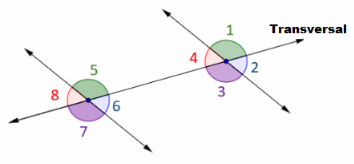# Math in Focus Grade 7 Chapter 6 Lesson 6.3 Answer Key Alternate Interior, Alternate Exterior, and Corresponding Angles

Practice the problems of Math in Focus Grade 7 Workbook Answer Key Chapter 6 Lesson 6.3 Alternate Interior, Alternate Exterior, and Corresponding Angles to score better marks in the exam.

## Math in Focus Grade 7 Course 2 B Chapter 6 Lesson 6.3 Answer Key Alternate Interior, Alternate Exterior, and Corresponding Angles

### Math in Focus Grade 7 Chapter 6 Lesson 6.3 Guided Practice Answer Key

Hands-On Activity

Materials:

• protractor

Explore The Angles Formed By Parallel Lines And A Transversal Using A Protractor

Work in pairs.

Step 1.
On a piece of paper, draw a pair of parallel lines, $$\overleftrightarrow{A B}$$ and $$\overleftrightarrow{C D}$$, as shown. Draw a transversal $$\overleftrightarrow{P Q}$$ that intersects the pair of parallel lines. Use a protractor to measure the angles in the diagram.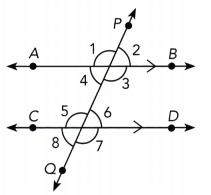Step 2.
Record your results in a table like the one shown.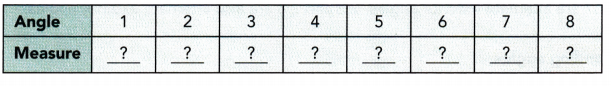Math Journal
What do you notice about the measures of these angle pairs: Alternate interior angles, alternate exterior angles, and corresponding angles? Make a conjecture about the angle measures for each pair.

Math Journal
Compare the conjecture that you have made for corresponding angles, alternate interior angles, and alternate exterior angles with the conjectures made by other students. What do you observe?

When two parallel lines are cut by a transversal,

• the alternate interior angles are always congruent (alt. int. ∠s, || lines).
• the alternate exterior angles are always congruent (alt. ext. ∠s, || lines).
• the corresponding angles are always congruent (corr. ∠s, || lines).

Use the diagram at the right to complete the following questions.

Question 1.
$$\overleftrightarrow{\mathrm{AD}}$$, $$\overleftrightarrow{\mathrm{EH}}$$, $$\overleftrightarrow{\mathrm{XY}}$$, and $$\overleftrightarrow{\mathrm{WZ}}$$ are straight lines and $$\overleftrightarrow{\mathrm{XY}}$$ is parallel to $$\overleftrightarrow{\mathrm{WZ}}$$. Identify all the pairs of angles formed by the intersection of $$\overleftrightarrow{\mathrm{AD}}$$ with $$\overleftrightarrow{\mathrm{XY}}$$ and $$\overleftrightarrow{\mathrm{WZ}}$$.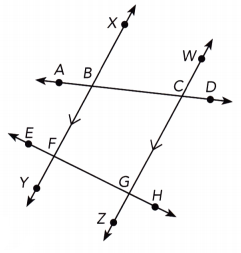$$\overline{X Y}$$ || $$\overline{W Z}$$ a) We are given:
∠ABX and ∠BCW Pairs of corresponding angles:
∠XBC and ∠WCD
∠EFB and ∠FGC
∠BFG and ∠CGH
∠XBC and ∠BCG b) Alternate interior angles:
∠FBC and ∠BCW

a) Corresponding angles: ∠ABX and ∠BCW;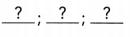∠XBC and ∠WCD
∠EFB and ∠FGC
∠BFG and ∠CGH

b) Alternate interior angles: ∠XBC and ∠BCG;∠FBC and ∠BCW

Question 2.
Name another transversal of the parallel lines in the diagram.
$$\overline{X Y}$$ || $$\overline{W Z}$$ we are given
A transversal of the parallel lines $$\overline{X Y}$$ and $$\overline{W Z}$$ other than $$\overline{A D}$$ is $$\overline{E H}$$.
$$\overline{E H}$$

Question 3.
Identify one pair of each of the following angles formed by the intersection of $$\overleftrightarrow{\mathrm{EH}}$$ with $$\overleftrightarrow{\mathrm{XY}}$$ and $$\overleftrightarrow{\mathrm{WZ}}$$.
a) Corresponding angles
$$\overline{X Y}$$ || $$\overline{W Z}$$ We are given:
∠EFY and ∠FOZ a) Identify a pair of corresponding angles formed by the intersection of $$\overline{E H}$$ with $$\overline{X Y}$$ and $$\overline{W Z}$$:

b) Alternate interior angles
∠BFG and ∠FGZ b) Identify a pair of alterrate interior angles formed by the intersection of $$\overline{E H}$$ with $$\overline{X Y}$$ and $$\overline{W Z}$$:

c) Alternate exterior angles
∠EFY and ∠CGR c) Identity a pair of aiterrate exterior angles formed by the intersection of $$\overline{E H}$$ with $$\overline{X Y}$$ and $$\overline{W Z}$$:
a) ∠EFY and ∠FGZ
b) ∠BFG and ∠FGZ
c) ∠EFY and ∠CGH

Complete.

Question 4.
In the diagram, $$\overleftrightarrow{\mathrm{MN}}$$ is parallel to $$\overleftrightarrow{\mathrm{PQ}}$$. Find the measures of ∠1, ∠2, and ∠3.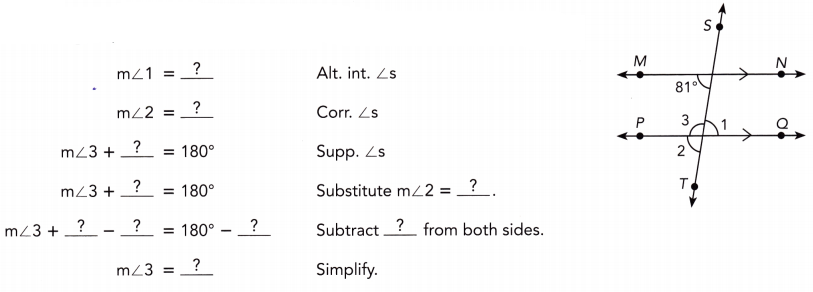$$\overline{M N}$$ || $$\overline{P Q}$$ We are given:
m∠1 = 81° Alternate interior ∠s:
m∠2 = 81° : Corresponding ∠s:
m∠3 + m∠2 = 180° Supplementary ∠s:
m∠3 + 81° = Substitute m∠2 = 81°:
m∠3 + 81° — 81° = 180° — 81° Subtract 81° from bothsides:
m∠3 = 99° simplify
81° ; 81° ; m∠2 ; 81° ; 81° ; 81° ; 81° ; 81° ; 99°

### Math in Focus Course 2B Practice 6.3 Answer Key

$$\overleftrightarrow{\mathrm{MN}}$$ is parallel to $$\overleftrightarrow{\mathrm{PQ}}$$. Identify each pair of angles as corresponding, alternate interior, alternate exterior angles, or none of the above.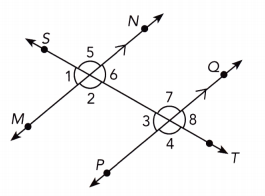Question 1.
∠3, ∠6
$$\overline{M N}$$ || $$\overline{P Q}$$ We are given:
∠3 and ∠6 are alternate interior angles because they lie inside the pair of parallel lines and on the opposite sides of the transversal.
Alternate interior angles

Question 2.
∠5, ∠7
$$\overline{M N}$$ || $$\overline{P Q}$$ We are given:
∠5 and ∠7 are corresponding angles because they lie in matching corners on same side of the transversal $$\overline{S T}$$.
corresponding angles

Question 3.
∠1, ∠2
$$\overline{M N}$$ || $$\overline{P Q}$$ We are given:
∠1 and ∠2 are neither alternate interior, nor alternate exterior or corresponding, therefore none of the above.
None of the above

Question 4.
∠1, ∠8
$$\overline{M N}$$ || $$\overline{P Q}$$ We are given:
∠1 and ∠8 are alternate exterior angles because they lie outside the pair of parallel lines and on the opposite sides of the transversal $$\overline{S T}$$.
Alternate exterior angles

Question 5.
∠8, ∠6
$$\overline{M N}$$ || $$\overline{P Q}$$ We are given:
∠8 and ∠6 are corresponding angles because they lie in matching corners on same side of the transversal $$\overline{S T}$$.
corresponding angles

Question 6.
∠4, ∠7
$$\overline{M N}$$ || $$\overline{P Q}$$ We are given:
∠4 and ∠7 are neither alternate interior, nor alternate exterior or corresponding, therefore none of the above.
None of the above

Question 7.
∠2, ∠7
$$\overline{M N}$$ || $$\overline{P Q}$$ We are given:
∠2 and ∠7 are alternate interior angles because they lie inside the pair of parallel lines and on the opposite sides of the transversal $$\overline{S T}$$.
Alternate interior angles

Question 8.
∠6, ∠7
$$\overline{M N}$$ || $$\overline{P Q}$$ We are given:
∠6 and ∠7 are neither alternate interior, nor alternate exterior or corresponding, therefore none of the above.
None of the above

$$\overleftrightarrow{\mathrm{AB}}$$ is parallel to $$\overleftrightarrow{\mathrm{CD}}$$. Use the diagram to answer the following.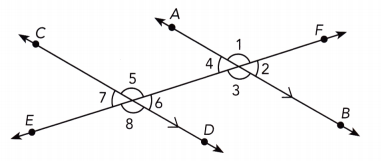Question 9.
Name two angles that have the same measure as ∠2.
$$\overline{A B}$$ || $$\overline{C D}$$ We are given:
m∠7 = m∠2 ∠2 and ∠7 are alternate exterior angles because they lie outside the pair of parallel lines and on the opposite sides of the transversal $$\overline{E F}$$.
m∠6 = m∠2 ∠2 and ∠6 are corresponding angles because they lie in matching corners on the same side of the transversal $$\overline{E F}$$.
∠6, ∠7

Question 10.
Name an angle that is supplementary to ∠6.
$$\overline{A B}$$ || $$\overline{C D}$$ We are given:
∠5, ∠8, ∠3, ∠1 We identify supplementary angles to ∠6:
∠5, ∠8, ∠3, ∠1

Question 11.
If m∠4 = 46°, find m∠5.
$$\overline{A B}$$ || $$\overline{C D}$$ We are given:
m∠6 = m∠4 Alternate interior ∠s:
m∠6 = 46° substitute:
m∠5 + m∠6 = 180° supplementary ∠s:
m∠5 + 46° = 180° substitute
m∠5 + 46° – 46° = 180° – 46° subtract 46° from both sides
m∠5 = 134° simplify
134°

Question 12.
If m∠1 = 131°, find m∠7.
$$\overline{A B}$$ || $$\overline{C D}$$ We are given:
Corresponding ∠s:
m∠5 = m∠1 corresponding ∠s:
m∠5 = 131° Substitute:
m∠5 + m∠7 = 180° Supplementary ∠s:
131° + m∠7 = 180° Substitute:
131° + m∠7 – 131° = 180° – 131° Subtract 131° from both sides:
m∠7 = 49° Simplify:
49°

Find the measure of each numbered angle.

Question 13.
$$\overleftrightarrow{M N}$$ is parallel to $$\overleftrightarrow{P Q}$$.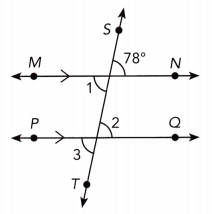$$\overline{M N}$$ || $$\overline{P Q}$$ We are given:
m∠1 = 78° vertical ∠s:
m∠1 = m∠2 Alternate interior ∠s:
m∠2 = 78° Substitute:
m∠3 = m∠2 vertical ∠s:
m∠3 = 78° Substitute:
m∠1 = 78° ;m∠2 = 78° ; m∠3 = 78°

Question 14.
$$\overleftrightarrow{M N}$$ is parallel to $$\overleftrightarrow{P Q}$$.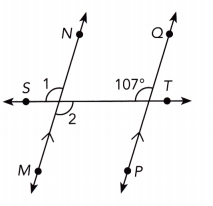$$\overline{M N}$$ || $$\overline{P Q}$$ We are given:
m∠1 = 107° vertical ∠s:
m∠1 = m∠2 corresponding ∠s:
m∠1 = m∠2 vertical ∠s:
m∠2 = 107° Substitute:
m∠1 = 107° ;m∠2 = 107°

Question 15.
$$\overleftrightarrow{P Q}$$ is parallel to $$\overleftrightarrow{R S}$$.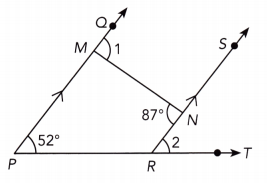$$\overline{P Q}$$ || $$\overline{R S}$$ We are given:
m∠1 = 87° Alternate interior ∠s:
m∠2 = 52° corresponding ∠s:
m∠1 = 87° ; m∠2 = 52°

Question 16.
$$\overleftrightarrow{P Q}$$ is parallel to $$\overleftrightarrow{R S}$$.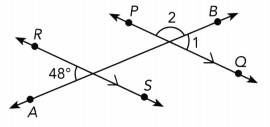$$\overline{P Q}$$ || $$\overline{R S}$$ We are given:
m∠1 = 48° Alternate exterior ∠s:
m∠1 + m∠2 = 180° supplementary ∠s:
48° + m∠2 = 180° substitute
48° + m∠2 – 48° = 180° – 48° subtract 48° from both sides
m∠2 = 132° simplify
m∠1 = 48° ; m∠2 = 132°

Question 17.
$$\overleftrightarrow{A B}$$ is parallel to $$\overleftrightarrow{C D}$$ and $$\overleftrightarrow{M N}$$ is parallel to $$\overleftrightarrow{P Q}$$.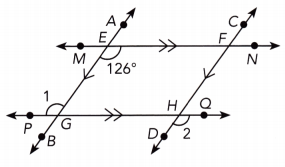$$\overline{M N}$$ || $$\overline{P Q}$$ We are given:
m∠1 = 126° Alternate interior ∠s:(transversal $$\overline{A B}$$)
$$\overline{A B}$$ || $$\overline{C D}$$ we are given
m∠2 = m∠1 Alternate exterior ∠s:(transversal $$\overline{P Q}$$)
m∠2 = 126° substitute
m∠1 = 126° ; m∠2 = 126°

Question 18.
$$\overleftrightarrow{A B}$$ is parallel to $$\overleftrightarrow{C D}$$ and $$\overleftrightarrow{M N}$$ is parallel to $$\overleftrightarrow{P Q}$$.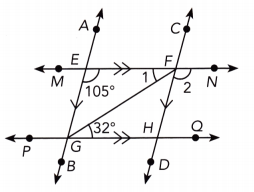$$\overline{M N}$$ || $$\overline{P Q}$$ We are given:
m∠1 = 32° Alternate interior ∠s:(transversal $$\overline{G F}$$)
$$\overline{A B}$$ || $$\overline{C D}$$ we are given
m∠2 = 105° corresponding ∠s:(transversal $$\overline{M N}$$)
m∠1 = 32° ; m∠2 = 105°

Find the value of each variable.

Question 19.
$$\overleftrightarrow{P Q}$$ is parallel to $$\overleftrightarrow{R S}$$.$$\overline{P Q}$$ || $$\overline{R S}$$ We are given:
a = 142 Alternate interior ∠s (transversal $$\overline{T U}$$):
a = 142
2b° + a° = 180° Supplementary ∠s
2b° + 142° = 180° Substitute:
2b° + 142° — 142° = 180° — 142° Subtract 142° from both sides:
2b° = 38°
$$\frac{2 b^{\circ}}{2}$$ = $$\frac{38^{\circ}}{2}$$ Divide by 2:
b = 19 simplify
a = 142
b = 19

Question 20.
$$\overleftrightarrow{P Q}$$ is parallel to $$\overleftrightarrow{R S}$$.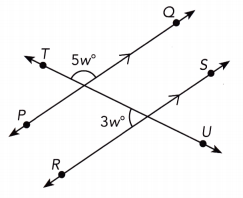$$\overline{P Q}$$ || $$\overline{R S}$$ We are given:
Let’s note by X the intersection between the lines $$\overline{T U}$$ and $$\overline{R S}$$.
m∠TXS = Corresponding ∠s (transversal $$\overline{T U}$$):
m∠TXS + m∠TXR = 180° Supplementary ∠s
5w° + 3w° = 180° Substitute:
8w° = 180° Simplify:
$$\frac{8 w^{\circ}}{8}$$ = $$\frac{180^{\circ}}{8}$$ Divide by 8
w = 22.5° simplify
w = 22.5°

MathJournal
Determine whether $$\overleftrightarrow{A B}$$ is parallel to $$\overleftrightarrow{C D}$$. Use the fact that two lines are parallel if a pair of corresponding angles formed by a transversal are congruent. Explain your answer.

Question 21.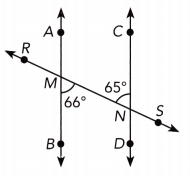m∠BMN = 66° ∠BMN and ∠MNC are alternate interior ∠s We have:
m∠BMN ≠ m∠MNC As 66° ≠ 65°, we have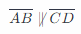This means the lines $$\overline{A B}$$ and $$\overline{C D}$$ are not parallel:Question 22.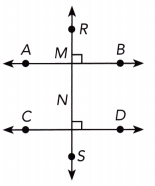m∠MND = 90° ∠RMB and ∠MND are corresponding ∠s. We have:
m∠MND = 90°
m∠RMB = m∠MND We get:
$$\overline{A B}$$ = $$\overline{C D}$$ This means the lines $$\overline{A B}$$ and $$\overline{C D}$$ are parallel:
$$\overline{A B}$$ || $$\overline{C D}$$

Question 23.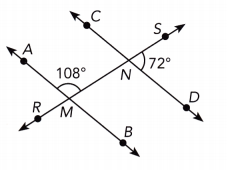m∠AMN + m∠NMB = 180° Supptementary ∠s
108° + m∠NMB = 180° Substitute:
108° + m∠NMB — 108° = 180° — 108° Subtract 108° from both sides:
m∠NMB = 72° Simplify:
m∠NMB = 72° ∠SND and ∠NMB are corresponding ∠s. We have:
m∠SND = m∠NMB we got
$$\overline{A B}$$ = $$\overline{C D}$$ This means the lines $$\overline{A B}$$ and $$\overline{C D}$$ are parallel
$$\overline{A B}$$ || $$\overline{C D}$$

$$\overline{M N}$$ is parallel to $$\overline{P Q}$$. Find each unknown angle measure.

Question 24.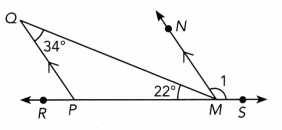m∠QMN = m∠PQM AS $$\overline{M N}$$ || $$\overline{M N}$$, ∠PQM and ∠QMN are alternate interior ∠s. We hava
m∠QMN = 34° Substitute
∠RMQ + m∠QMN + m∠1 = 180° ∠RMS straight angle
22° + 34° + m∠1 = 180°
56° + m∠1 = 180° Simplify
56° + m∠1 – 56° = 180° – 56° subtract 56° from both sides
m∠1 = 124° simplify
m∠1 = 124°

Question 25.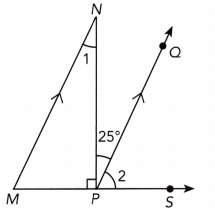m∠1 = m∠NPQ As $$\overline{M N}$$ and $$\overline{P Q}$$, ∠1 and ∠NPQ are alternate interior ∠s. We have:
m∠1 = 25° Substitute:
m∠MPN + m∠NPQ + m∠2 = 180° ∠MPS straight:
90° + 25° + m∠2 = 180° Substitute:
115° + m∠2 = 180° Simplify:
115° + m∠2 – 115° = 180° – 115° subtract 115° from both sides
m∠2 = 65° simplify
m∠1 = 25°
m∠2 = 65°

Question 26.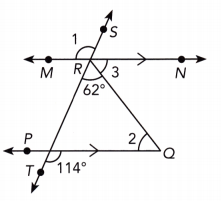m∠TRN = 114° As $$\overline{M N}$$ || $$\overline{P Q}$$, a pair of corresponding angles is:
62° + m∠3 = 114° Substitute:
62° + m∠3 – 62° = 114° — 62° Subtract 62° from both sides:
m∠3 = 52° simplify
m∠2 = m∠3 alternate interior ∠s
m∠2 = 52°
m∠1 = 114° Alternate exterior ∠s
m∠1 = 114°
m∠2 = 52°
m∠3 = 52°

Question 27.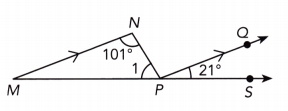m∠NPQ = m∠MNP As $$\overline{M N}$$ || $$\overline{P Q}$$, ∠MNP and ∠NPQ are alternate interior angles
m∠NPQ = 101° Substitute:
m∠1 + m∠NPQ + m∠QPS = 180° ∠MPS is a straight line
m∠1 + 101° + 21° = 180° substitute
m∠1 + 122° = 180° simplify
m∠1 + 122° – 122° = 180° – 122° subtract 122° from both sides
m∠1 = 58° simplify
m∠1 = 58°

Question 28.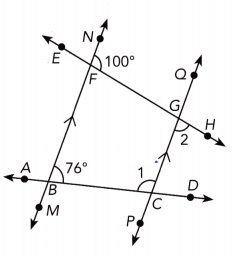m∠DCQ = m∠DBN As $$\overline{M N}$$ || $$\overline{P Q}$$, a pair of a corresponding angles is :
m∠DCQ = 76° Substitute
m∠1 + m∠DCQ = 180° ∠ACD is straight
m∠1 + 76° = 180° substitute
m∠1 + 76° – 76° = 180° – 76° subtract 76° from both sides
m∠1 = 104° simplify
m∠MFH + m∠HFN = 180° m∠MFH straight line
m∠MFH + 100° = 180° substitute
m∠MFH + 100° – 100° = 180° Subtract 100° from both sides:
m∠MFH = 80° Simplify:
m∠PGH = m∠MFH ∠MFH and ∠PGH corresponding angles:
m∠2 = 80° Substitute:
m∠1 = 104°
m∠2 = 80°

Question 29.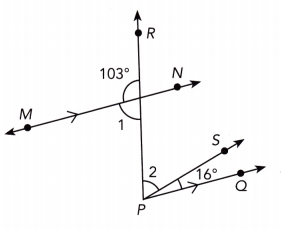m∠1 + 103° = 180° Straight ∠
m∠1 + 103° — 103° = 180° — 103° Subtract 103° from both sides:
m∠1 = 77° Simplify:
m∠1 = m∠2 + 16° As $$\overline{M N}$$ || $$\overline{P Q}$$, a pair of alternate interior angles is:
77° = m∠2 + 16° Substitute:
77° – 16° = m∠2 + 16 — 16° Subtract 16° from both sides:
m∠2 = 61° Simplify:
m∠1 = 77°
m∠2 = 61°

$$\overline{\mathbf{A B}}$$ is parallel to $$\overline{\mathbf{C D}}$$. Find the value of x.

Question 30.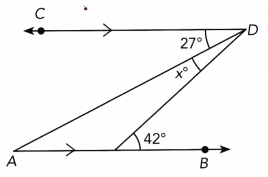27° + x° = 42 As $$\overline{A B}$$ || $$\overline{C D}$$, a pair of alternate interior angles is:
27° + x° — 27° = 42° — 27° Subtract 27° from both sides:
x° = 15° Simplify:
x = 15

Question 31.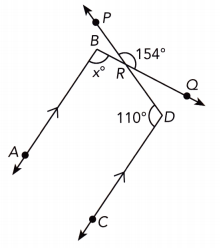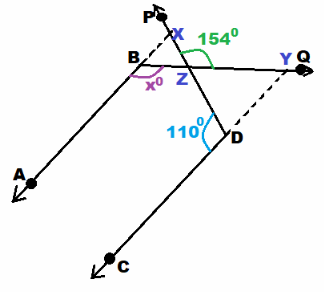we are given
Let X be the intersection of the Lines $$\overline{A B}$$ and $$\overline{P D}$$, Y be the intersection of the lines $$\overline{C D}$$ and $$\overline{B Q}$$ and Z be the intersection of the lines $$\overline{P D}$$ || $$\overline{B Q}$$.
110° + m∠PDY = 180° ∠CDY is straight
110° + m∠PDY = 180° – 110° subtract 110° from both sides
m∠PDY = 70° simplify
m∠DZY + 154° = 180° ∠PZD is straight
m∠DZY + 154° – 154 = 180° — 134° Subtract 154° from both sides:
m∠DZY = 26° Simplify:
m∠DZY + m∠PDY + m∠BYD = 180° Sum of the angles in ∆ZDY:
26° + 70° + m∠BYD = 180° Substitute:
96° + m∠BYD = 180° Simplify:
96° + m∠BYD – 96° = 180° — 96° Subtract 96° from both sides:
m∠BYD = 84° Simplify:
m∠BYD + m∠DYQ = 180° ∠BYQ is straight:
84° + m∠DYQ = 180° Substitute:
84° + m∠DYQ – 84° = 180° – 84° Subtract 84° from both sides:
m∠DYQ = 96° Simplify:
m∠ABQ = m∠DYQ As $$\overline{A B}$$ || $$\overline{C D}$$, a pair of corresponding angles is:
x° = 96° Substitute:
x = 96

Question 32.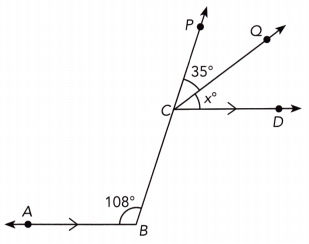m∠BCD = m∠ABC As $$\overline{A B}$$ || $$\overline{C D}$$, a pair of alternate interior angles is:
m∠BCD = 108° Substitute:
m∠BCD + m∠DCQ + m∠QCP = 180° ∠BCP is straight:
108° + x° + 35° = 180° Substitute:
143° + x° = 180° Simplify:
143° + x° — 143° = 180° – 143° Substitute:
x = 37 Simplify:
x = 37

Question 33.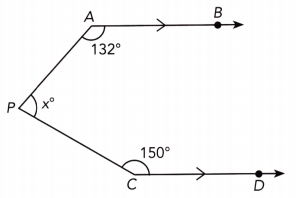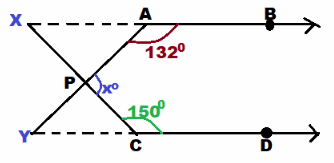we are given
Let X be the intersection of the lines $$\overline{A B}$$ and $$\overline{P C}$$, Y be the intersection of the lines $$\overline{C D}$$ and $$\overline{A P}$$.
150° + m∠XCY = 180° ∠YCD is straight:
150° + m∠XCY — 150° = 180° — 150° Subtract 150° from both sides:
m∠XCY = 30° Simplify:
m∠XAP + 132° = 180° ∠XAB is straight:
m∠XAP = 48° simplify
m∠XAP = m∠PYC As $$\overline{A B}$$ || $$\overline{C D}$$, a pair of alternate interior angles is:
m∠PYC = 48° Substitute:
m∠PYC + m∠XCY + m∠YPC = Sum of the ∠s in a triangle:
48° + 30° + m∠YPC = 180° Substitute:
78° + m∠YPC = Simplify:
78° + m∠YPC — 78° = 180° — 78° Subtract 78° from both sides:
m∠YPC = 102° Simplify:
m∠YPC + x° = 180° ∠APY straight:
102 + x° = 180° Substitute:
102° + x° – 102° = 180° — 102 Subtract 102° from both sides:
x° = 78° simplify
x = 78

Question 34.
In the diagram below, $$\overleftrightarrow{M N}$$ is parallel to $$\overleftrightarrow{P Q}$$. m∠1 = (x + 28)° and m∠2 = (3x + 14)°. Write and solve an equation to find the measures of ∠1 and ∠2.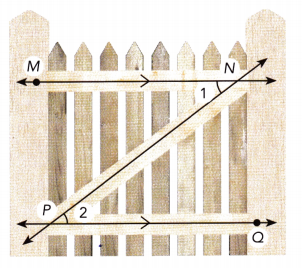m∠1 = m∠2 As $$\overline{M N}$$ || $$\overline{P Q}$$, a pair of alternate interior angles is:
x + 28 = 3x + 11 substitute
x + 28 – x = 3x + 14 – x Subtract x from both sides:
28 = 2x + 14 simplify
28 — 14 = 2x + 14 — 14 Subtract 14 from both sides:
2x = 14 Simplify:
$$\frac{2 x}{2}$$ = $$\frac{14}{2}$$ Divide by 2:
x = 7 Simplify:
m∠1 = m∠2 = (7 + 28)° = 35° Determine m∠1, m∠2:
x + 28 = 3x + 14
m∠1 = 35° ;m∠2 = 35°

Question 35.
Math Journal
In a plane, if a line is perpendicular to one of two parallel lines, is it also perpendicular to the other? Explain your reasoning.
$$\overline{A B}$$ || $$\overline{C D}$$ We are given:
$$\overline{E F}$$ ⊥ $$\overline{A B}$$
m∠EMB = 90°: $$\overline{E F}$$ ⊥ $$\overline{A B}$$
m∠MND = m∠EMB As $$\overline{A B}$$ || $$\overline{C D}$$, a pair of corresponding angles is:
m∠MND = 90° Substitute:
$$\overline{E F}$$ ⊥ $$\overline{C D}$$ m∠MND = 90°
yes

Question 36.
The diagram below contains examples of parallel lines cut by transversals. Line MN is parallel to line PQ and line AB is parallel to line CO.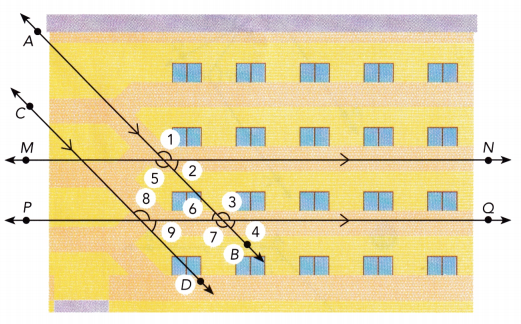a) Name two pairs of corresponding angles.
∠1 and ∠3 a) As $$\overline{A B}$$ || $$\overline{P Q}$$, pairs of corresponding angles are (transversal $$\overline{A B}$$):
∠2 and ∠4
∠5 and ∠7

b) Name all the angles that have the same measure as ∠1.
m∠5 = m∠1 b) vertical ∠s:
m∠3 = m∠1 corresponding ∠s:
m∠8 = m∠3 = m∠1
m∠7 = m∠5 = m∠1
a) ∠1 and ∠3
∠2 and ∠4
∠5 and ∠7
b) ∠1, ∠3, ∠5, ∠7 = ∠8

Question 37.
The two mirrors used in a periscope are parallel to each other as shown. m∠1 = 3x°, m∠2 = (60 – x)°, and m∠3 = 90°. Write and solve an equation to find the value of x. Then find the measure of ∠4.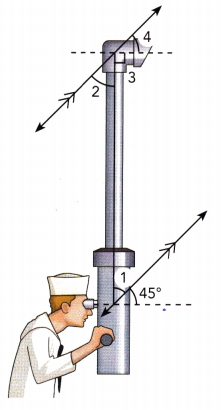m∠1 = 3x° We are given:
m∠2 = (60 – x)°
m∠3 = 90°
m∠1 = m∠2 Alternate interior ∠s
3x° = (60 – x)°
3x = 60 — x Substitute:
3x + x = 60 – x + x Add x to both sides:
4x = 60 Simplify:
$$\frac{4 x}{4}$$ = $$\frac{60}{4}$$ Divide by 4:
x = 15 Simplify:
m∠2 + m∠3 + m∠4 = 180° Straight ∠:
Substitute:
(60 – x)° + 90° + m∠4 = 180°
(60 – 15)° + 90° + m∠4 = 180°
45° + 90° + m∠4 = 180°
135° + m∠4 = 180° Simplify:
135° + m∠4 – 135° = 180° — 135° Subtract 135° from both sides:
m∠4 = 45° Simplify:
x = 15
m∠4 = 45°

Question 38.
MathJournal
Use a diagram to illustrate each of the following: transversal, corresponding angles, alternate exterior angles, and alternate interior angles. Label your diagram and explain which angles are congruent.